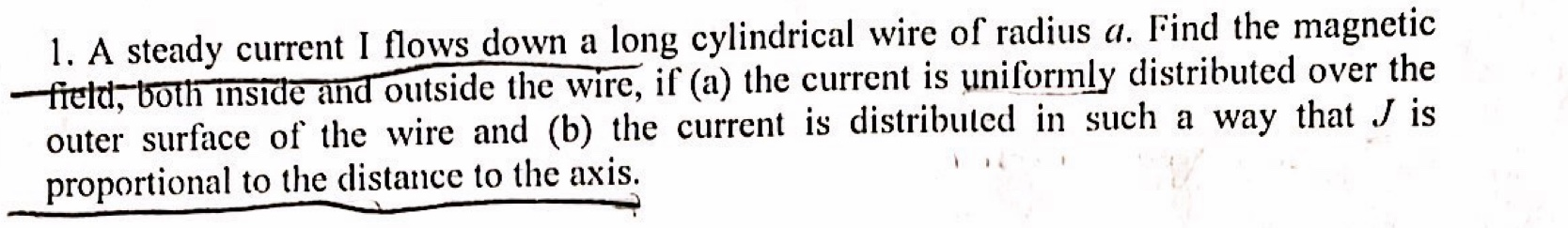# 1. A steady current I flows down a long cylindrical wire of radius a. Find the magneticfreld, both inside and outside the wire, if (a) the current is uniformly distributed over theouter surface of the wire and (b) the current is distributed in such a way that J isproportional to the distance to the axis

Questionhelp_outlineImage Transcriptionclose1. A steady current I flows down a long cylindrical wire of radius a. Find the magnetic freld, both inside and outside the wire, if (a) the current is uniformly distributed over the outer surface of the wire and (b) the current is distributed in such a way that J is proportional to the distance to the axis fullscreen
check_circle

Step 1

(a) Let the radius of amperian loop be r. For r < a, the magnetic field is zero. Outside the loop, the magnetic field is given by,

Step 2

(b) Current density J is proportional to r. Therefore, the current is,

Step 3

For r < a, the curre...

### Want to see the full answer?

See Solution

#### Want to see this answer and more?

Solutions are written by subject experts who are available 24/7. Questions are typically answered within 1 hour.*

See Solution
*Response times may vary by subject and question.
Tagged in

### Science